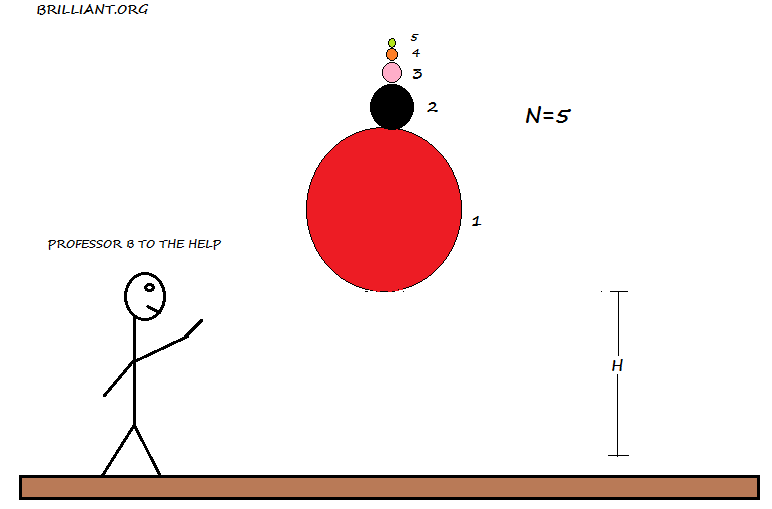# Bouncy balls put on top of each otherBouncy balls are stacked on top of each other with decreasing mass as shown In the figure above. They are configured such that the one on the bottom is at a height of $H$ above the ground. The figure above shown for $n=5$, but we can increase the number of ball to our liking.

After the balls are released, and the first ball touches the ground, each ball bounces off its neighboring ball. If $v_{n}$ is the speed of the $nth$ ball right after it bounces of its adjacent ball. What is the ratio of the speed of the $20th$ ball right after it bounces to the $5th$ ball right after it bounces.

Simply put what is $\large{\frac { { v }_{ 20 } }{ { v }_{ 5 } }}$

Details and assumptions

All the collisions that take place are elastic collisions.

Assume ${ M }_{ 1 }\gg { M }_{ 2 }\gg { M }_{ 3 }\gg ........{ M }_{ n }$.

×